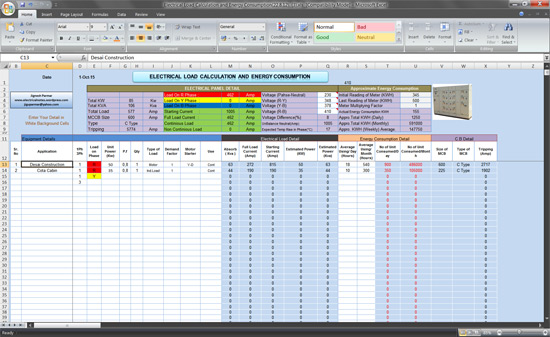# Calculul sarcinii electrice și a consumului de energie (foaie de calcul MS Excel)This MS Excel spreadsheet calculate following

• Continuous and non-continuous electrical load of panel
• Total energy consumption (KWH) in daily/monthly of panel
• Size of MCB of each branch circuit of panel
• Voltage / Voltage difference of each phase
• Unbalanced load in neutral wire
• Expected temperature rise in each phase
• Size / type / tripping setting of main MCCB